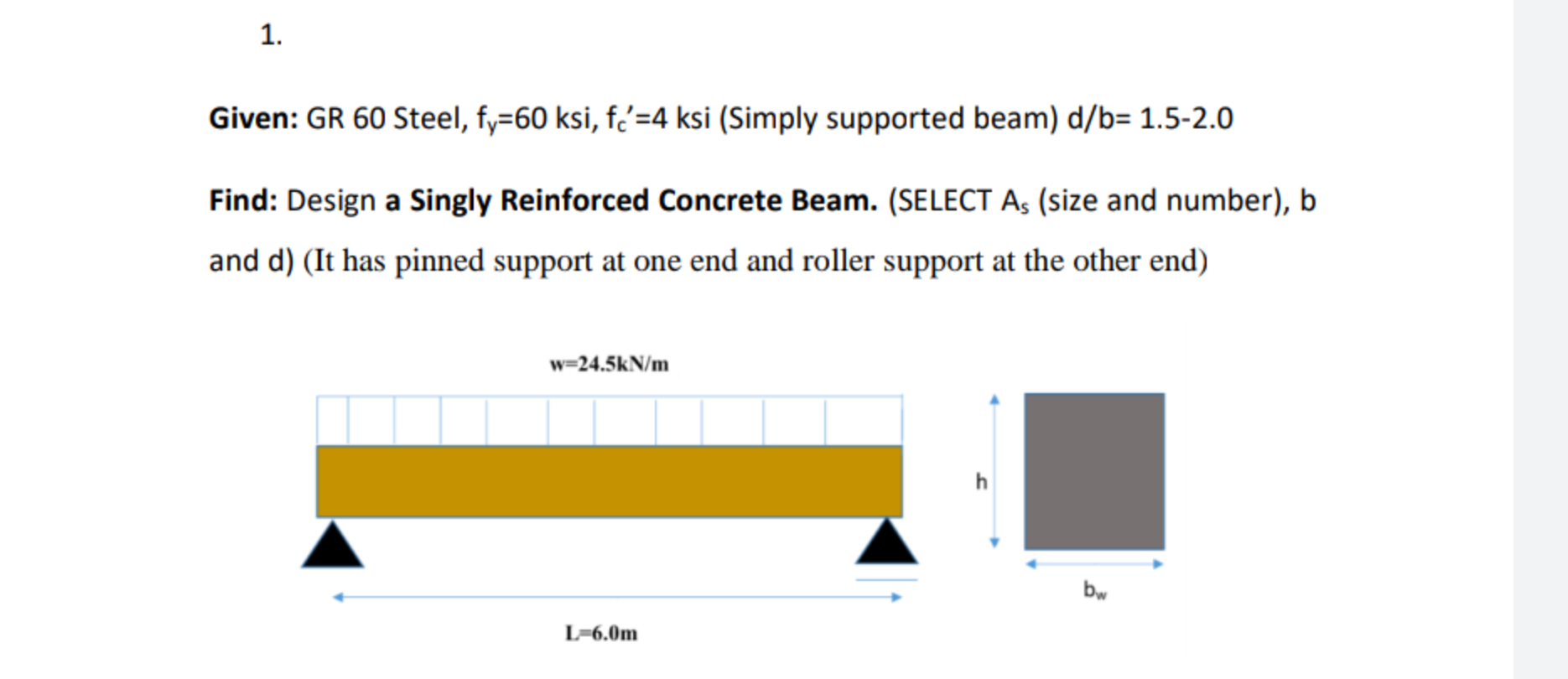Home / Expert Answers / Civil Engineering / use-turkish-standard-from-ts-500-given-gr-60-steel-f-y-60-mathrm-ksi-mathrm-f-mathr-pa816

# (Solved): use turkish standard from ts 500 Given: GR 60 Steel, $$f_{y}=60 \mathrm{ksi}, \mathrm{f}_{\mathr ...use turkish standard from ts 500 Given: GR 60 Steel, \( f_{y}=60 \mathrm{ksi}, \mathrm{f}_{\mathrm{c}}{ }^{\prime}=4 \mathrm{ksi}$$ (Simply supported beam) $$\mathrm{d} / \mathrm{b}=1.5-2.0$$ Find: Design a Singly Reinforced Concrete Beam. (SELECT A $$A_{s}$$ (size and number), b and d) (It has pinned support at one end and roller support at the other end)

We have an Answer from Expert